# NCERT solutions for Mathematics Exemplar Class 8 chapter 11 - Mensuration [Latest edition]

#### Chapters## Chapter 11: Mensuration

Exercise
Exercise [Pages 344 - 360]

### NCERT solutions for Mathematics Exemplar Class 8 Chapter 11 Mensuration Exercise [Pages 344 - 360]

#### Choose the correct alternative:

Exercise | Q 1 | Page 344

A cube of side 5 cm is painted on all its faces. If it is sliced into 1 cubic centimetre cubes, how many 1 cubic centimetre cubes will have exactly one of their faces painted?

• 27

• 42

• 54

• 142

Exercise | Q 2 | Page 345

A cube of side 4 cm is cut into 1 cm cubes. What is the ratio of the surface areas of the original cubes and cut-out cubes?

• 1 : 2

• 1 : 3

• 1 : 4

• 1 : 6

Exercise | Q 3 | Page 345

A circle of maximum possible size is cut from a square sheet of board. Subsequently, a square of maximum possible size is cut from the resultant circle. What will be the area of the final square?

• 3/4 of original square

• 1/2 of original square

• 1/4 of original square

• 2/3 of original square

Exercise | Q 4 | Page 345

What is the area of the largest triangle that can be fitted into a rectangle of length l units and width w units?

• (lw)/2

• (lw)/3

• (lw)/6

• (lw)/4

Exercise | Q 5 | Page 345

If the height of a cylinder becomes 1/4 of the original height and the radius is doubled, then which of the following will be true?

• Volume of the cylinder will be doubled

• Volume of the cylinder will remain unchanged

• Volume of the cylinder will be halved

• Volume of the cylinder will be 1/4 of the original volume

Exercise | Q 6 | Page 346

If the height of a cylinder becomes 1/4 of the original height and the radius is doubled, then which of the following will be true?

• Curved surface area of the cylinder will be doubled

• Curved surface area of the cylinder will remain unchanged

• Curved surface area of the cylinder will be halved

• Curved surface area will be 1/4 of the original curved surface

Exercise | Q 7 | Page 346

If the height of a cylinder becomes 1/4 of the original height and the radius is doubled, then which of the following will be true?

• Total surface area of the cylinder will be doubled

• Total surface area of the cylinder will remain unchanged

• Total surface of the cylinder will be halved

• None of the above

Exercise | Q 8 | Page 346

The surface area of the three coterminus faces of a cuboid are 6, 15 and 10 cm2 respectively. The volume of the cuboid is ______.

• 30 cm3

• 40 cm3

• 20 cm3

• 35 cm3

Exercise | Q 9 | Page 346

A regular hexagon is inscribed in a circle of radius r. The perimeter of the regular hexagon is ______.

• 3r

• 6r

• 9r

• 12r

Exercise | Q 10 | Page 346

The dimensions of a godown are 40 m, 25 m and 10 m. If it is filled with cuboidal boxes each of dimensions 2 m × 1.25 m × 1 m, then the number of boxes will be ______.

• 1800

• 2000

• 4000

• 8000

Exercise | Q 11 | Page 347

The volume of a cube is 64 cm3. Its surface area is ______.

• 16 cm2

• 64 cm2

• 96 cm2

• 128 cm2

Exercise | Q 12 | Page 347

If the radius of a cylinder is tripled but its curved surface area is unchanged, then its height will be ______.

• Tripled

• Constant

• One-sixth

• One-third

Exercise | Q 13 | Page 347

How many small cubes with edge of 20 cm each can be just accommodated in a cubical box of 2 m edge?

• 10

• 100

• 1000

• 10000

Exercise | Q 14 | Page 347

The volume of a cylinder whose radius r is equal to its height is ______.

• 1/4 pir^3

• (pir^3)/32

• pir^3

• r^3/8

Exercise | Q 15 | Page 347

The volume of a cube whose edge is 3x is ______.

• 27x3

• 9x3

• 6x3

• 3x3

Exercise | Q 16 | Page 347

The figure ABCD is a quadrilateral in which AB = CD and BC = AD. Its area is ______.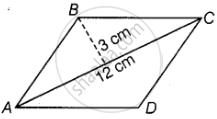• 72 cm2

• 36 cm2

• 24 cm2

• 18 cm2

Exercise | Q 17 | Page 347

What is the area of the rhombus ABCD below if AC = 6 cm, and BE = 4cm?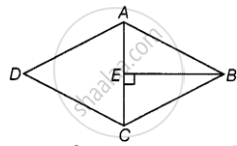• 36 cm2

• 16 cm2

• 24 cm2

• 13 cm2

Exercise | Q 18 | Page 348

The area of a parallelogram is 60 cm2 and one of its altitudes is 5 cm. The length of its corresponding side is ______.

• 12 cm

• 6 cm

• 4 cm

• 2 cm

Exercise | Q 19 | Page 348

The perimeter of a trapezium is 52 cm and its each non-parallel side is equal to 10 cm with its height 8 cm. Its area is ______.

• 124 cm2

• 118 cm2

• 128 cm2

• 112 cm2

Exercise | Q 20 | Page 348

Area of a quadrilateral ABCD is 20 cm2 and perpendiculars on BD from opposite vertices are 1 cm and 1.5 cm. The length of BD is ______.

• 4 cm

• 15 cm

• 16 cm

• 18 cm

Exercise | Q 21 | Page 348

A metal sheet 27 cm long, 8 cm broad and 1 cm thick is melted into a cube. The side of the cube is ______.

• 6 cm

• 8 cm

• 12 cm

• 24 cm

Exercise | Q 22 | Page 348

Three cubes of metal whose edges are 6 cm, 8 cm and 10 cm respectively are melted to form a single cube. The edge of the new cube is ______.

• 12 cm

• 24 cm

• 18 cm

• 20 cm

Exercise | Q 23 | Page 348

A covered wooden box has the inner measures as 115 cm, 75 cm and 35 cm and thickness of wood as 2.5 cm. The volume of the wood is ______.

• 85,000 cm3

• 80,000 cm3

• 82,125 cm3

• 84,000 cm3

Exercise | Q 24 | Page 348

The ratio of radii of two cylinders is 1: 2 and heights are in the ratio 2:3. The ratio of their volumes is ______.

• 1:6

• 1:9

• 1:3

• 2:9

Exercise | Q 25 | Page 349

Two cubes have volumes in the ratio 1:64. The ratio of the area of a face of first cube to that of the other is ______.

• 1:4

• 1:8

• 1:16

• 1:32

Exercise | Q 26 | Page 349

The surface areas of the six faces of a rectangular solid are 16, 16, 32, 32, 72 and 72 square centimetres. The volume of the solid, in cubic centimetres, is ______.

• 192

• 384

• 480

• 2592

Exercise | Q 27 | Page 349

Ramesh has three containers.
(a) Cylindrical container A having radius r and height h,
(b) Cylindrical container B having radius 2r and height 1/2 h, and
(c) Cuboidal container C having dimensions r × r × h
The arrangement of the containers in the increasing order of their volumes is ______.

• A, B, C

• B, C, A

• C, A, B

• Cannot be arranged

Exercise | Q 28 | Page 349

If R is the radius of the base of the hat, then the total outer surface area of the hat is ______.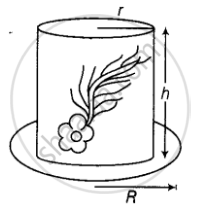• pir(2h + R)

• 2pir(h + R)

• 2pirh + pir^2

• None of these

#### Fill in the blanks:

Exercise | Q 29 | Page 349

A cube of side 4 cm is painted on all its sides. If it is sliced in 1 cubic cm cubes, then number of such cubes that will have exactly two of their faces painted is ______.

Exercise | Q 30 | Page 349

A cube of side 5 cm is cut into 1 cm cubes. The percentage increase in volume after such cutting is ______.

Exercise | Q 31 | Page 349

The surface area of a cuboid formed by joining two cubes of side a face to face is ______.

Exercise | Q 32 | Page 350

If the diagonals of a rhombus get doubled, then the area of the rhombus becomes __________ its original area.

Exercise | Q 33 | Page 350

If a cube fits exactly in a cylinder with height h, then the volume of the cube is ______ and surface area of the cube is ______.

Exercise | Q 34 | Page 350

The volume of a cylinder becomes ______ the original volume if its radius becomes half of the original radius.

Exercise | Q 35 | Page 350

The curved surface area of a cylinder is reduced by ______ percent if the height is half of the original height.

Exercise | Q 36 | Page 350

The volume of a cylinder which exactly fits in a cube of side a is ______.

Exercise | Q 37 | Page 350

The surface area of a cylinder which exactly fits in a cube of side b is ______.

Exercise | Q 38 | Page 350

If the diagonal d of a quadrilateral is doubled and the heights h1 and h2 falling on d are halved, then the area of quadrilateral is ______.

Exercise | Q 39 | Page 350

The perimeter of a rectangle becomes ______ times its original perimeter, if its length and breadth are doubled.

Exercise | Q 40 | Page 350

A trapezium with 3 equal sides and one side double the equal side can be divided into ______ equilateral triangles of ______ area.

Exercise | Q 41 | Page 350

All six faces of a cuboid are ______ in shape and of ______ area.

Exercise | Q 42 | Page 350

Opposite faces of a cuboid are ______ in area.

Exercise | Q 43 | Page 350

Curved surface area of a cylinder of radius h and height r is ______.

Exercise | Q 44 | Page 350

Total surface area of a cylinder of radius h and height r is ______.

Exercise | Q 45 | Page 350

Volume of a cylinder with radius h and height r is ______.

Exercise | Q 46 | Page 351

Area of a rhombus = 1/2 product of ______.

Exercise | Q 47 | Page 351

Two cylinders A and B are formed by folding a rectangular sheet of dimensions 20 cm × 10 cm along its length and also along its breadth respectively. Then volume of A is ______ of volume of B

Exercise | Q 48 | Page 351

In the above question, curved surface area of A is ______ curved surface area of B.

Exercise | Q 49 | Page 351

______ of a solid is the measurement of the space occupied by it.

Exercise | Q 50 | Page 351

______ surface area of room = area of 4 walls.

Exercise | Q 51 | Page 351

Two cylinders of equal volume have heights in the ratio 1:9. The ratio of their radii is ______.

Exercise | Q 52 | Page 351

Two cylinders of same volume have their radii in the ratio 1:6, then ratio of their heights is ______.

#### State whether the following statement is True or False:

Exercise | Q 53 | Page 351

The areas of any two faces of a cube are equal.

• True

• False

Exercise | Q 54 | Page 351

The areas of any two faces of a cuboid are equal.

• True

• False

Exercise | Q 55 | Page 351

The surface area of a cuboid formed by joining face to face 3 cubes of side x is 3 times the surface area of a cube of side x.

• True

• False

Exercise | Q 56 | Page 351

Two cuboids with equal volumes will always have equal surface areas.

• True

• False

Exercise | Q 57 | Page 351

The area of a trapezium become 4 times if its height gets doubled

• True

• False

Exercise | Q 58 | Page 351

A cube of side 3 cm painted on all its faces, when sliced into 1 cubic centimetre cubes, will have exactly 1 cube with none of its faces painted.

• True

• False

Exercise | Q 59 | Page 351

Two cylinders with equal volume will always have equal surface areas.

• True

• False

Exercise | Q 60 | Page 351

The surface area of a cube formed by cutting a cuboid of dimensions 2 × 1 × 1 in 2 equal parts is 2 sq.units.

• True

• False

Exercise | Q 61 | Page 351

Ratio of area of a circle to the area of a square whose side equals radius of circle is 1 : π.

• True

• False

#### Solve the following:

Exercise | Q 62 | Page 352

The area of a rectangular field is 48 m2 and one of its sides is 6m. How long will a lady take to cross the field diagonally at the rate of 20 m/minute?

Exercise | Q 63 | Page 352

The circumference of the front wheel of a cart is 3 m long and that of the back wheel is 4 m long. What is the distance travelled by the cart, when the front wheel makes five more revolutions than the rear wheel?

Exercise | Q 64 | Page 352

Four horses are tethered with equal ropes at 4 corners of a square field of side 70 metres so that they just can reach one another. Find the area left ungrazed by the horses.

Exercise | Q 65 | Page 352

The walls and ceiling of a room are to be plastered. The length, breadth and height of the room are 4.5 m, 3 m, and 350 cm respectively. Find the cost of plastering at the rate of Rs 8 per m2.

Exercise | Q 66.(i) | Page 352

Most of the sailboats have two sails, the jib and the mainsail. Assume that the sails are triangles. Find the total area sail of the sailboats to the nearest tenth.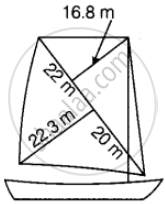Exercise | Q 66.(ii) | Page 352

Most of the sailboats have two sails, the jib and the mainsail. Assume that the sails are triangles. Find the total area sail of the sailboats to the nearest tenth.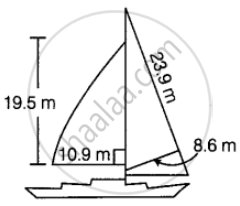Exercise | Q 66.(iii) | Page 352

Most of the sailboats have two sails, the jib and the mainsail. Assume that the sails are triangles. Find the total area sail of the sailboats to the nearest tenth.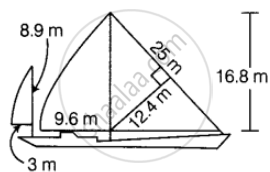Exercise | Q 67 | Page 352

The area of a trapezium with equal non-parallel sides is 168 m2. If the lengths of the parallel sides are 36 m and 20 m, find the length of the non-parallel sides.

Exercise | Q 68 | Page 352

Mukesh walks around a circular track of radius 14 m with a speed of 4 km/hr. If he takes 20 rounds of the track, for how long does he walk?

Exercise | Q 69 | Page 353

The areas of two circles are in the ratio 49:64. Find the ratio of their circumferences.

Exercise | Q 70 | Page 353

There is a circular pond and a footpath runs along its boundary. A person walks around it, exactly once keeping close to the edge. If his step is 66 cm long and he takes exactly 400 steps to go around the pond, find the diameter of the pond.

Exercise | Q 71 | Page 353

A running track has 2 semicircular ends of radius 63 m and two straight lengths. The perimeter of the track is 1000 m. Find each straight length.

Exercise | Q 72 | Page 353

Find the perimeter of the given figure.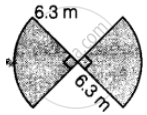Exercise | Q 73 | Page 353

A bicycle wheel makes 500 revolutions in moving 1 km. Find the diameter of the wheel.

Exercise | Q 74 | Page 353

A boy is cycling such that the wheels of the cycle are making 140 revolutions per hour. If the diameter of the wheel is 60 cm, calculate the speed in km/h with which the boy is cycling

Exercise | Q 75 | Page 353

Find the length of the largest pole that can be placed in a room of dimensions 12 m × 4 m × 3 m.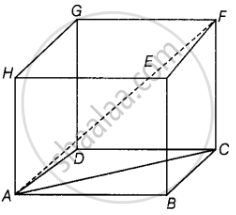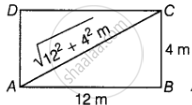Exercise | Q 76 | Page 354

Find the area of the following fields. All dimensions are in metres.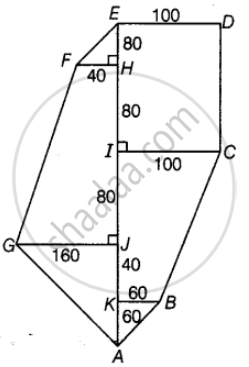Exercise | Q 77 | Page 354

Find the area of the following fields. All dimensions are in metres.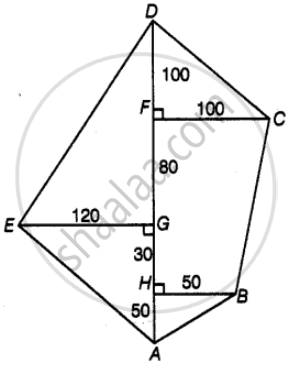Exercise | Q 78 | Page 354

Find the area of the shaded portion in the following figure.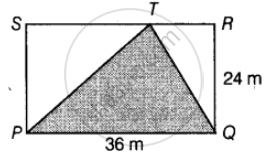Exercise | Q 79 | Page 354

Find the area of the shaded portion in the following figure.Exercise | Q 80 | Page 354

Find the area of the shaded portion in the following figure.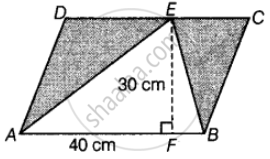Exercise | Q 81 | Page 354

Find the area of the shaded portion in the following figure.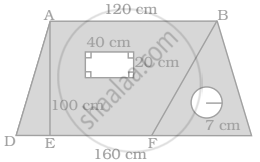Exercise | Q 82 | Page 355

Find the area of the shaded portion in the following figure.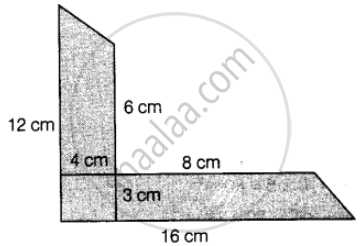Exercise | Q 83 | Page 354

Find the area of the shaded portion in the following figure.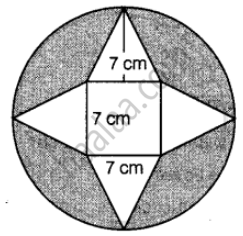Exercise | Q 84 | Page 355

Find the area of the shaded portion in the following figure.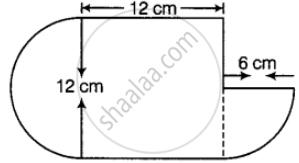Exercise | Q 85 | Page 355

Find the area of the shaded portion in the following figure.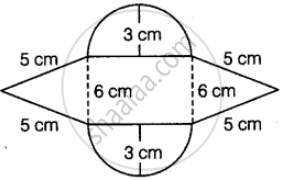Exercise | Q 86.(a) | Page 355

Find the volume of the given figure if volume = base area × height.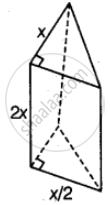Exercise | Q 86.(b) | Page 355

Find the volume of the given figure if volume = base area × height.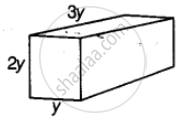Exercise | Q 86.(c) | Page 355

Find the volume of the given figure if volume = base area × height.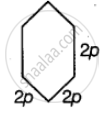Exercise | Q 87 | Page 355

A cube of side 5 cm is cut into as many 1 cm cubes as possible. What is the ratio of the surface area of the original cube to that of the sum of the surface areas of the smaller cubes?

Exercise | Q 88 | Page 356

A square sheet of paper is converted into a cylinder by rolling it along its side. What is the ratio of the base radius to the side of the square?

Exercise | Q 89 | Page 356

How many cubic metres of earth must be dug to construct a well 7 m deep and of diameter 2.8 m?

Exercise | Q 90 | Page 356

The radius and height of a cylinder are in the ratio 3:2 and its volume is 19,404 cm3. Find its radius and height.

Exercise | Q 91 | Page 356

The thickness of a hollow metallic cylinder is 2 cm. It is 70 cm long with outer radius of 14 cm. Find the volume of the metal used in making the cylinder, assuming that it is open at both the ends. Also find its weight if the metal weighs 8 g per cm3.

Exercise | Q 92.(a) | Page 356

Radius of a cylinder is r and the height is h. Find the change in the volume if the height is doubled.

Exercise | Q 92.(b) | Page 356

Radius of a cylinder is r and the height is h. Find the change in the volume if the height is doubled and the radius is halved.

Exercise | Q 92.(c) | Page 356

Radius of a cylinder is r and the height is h. Find the change in the volume if the height remains same and the radius is halved.

Exercise | Q 93 | Page 356

If the length of each edge of a cube is tripled, what will be the change in its volume?

Exercise | Q 94 | Page 356

A carpenter makes a box which has a volume of 13,400 cm3. The base has an area of 670 cm2. What is the height of the box?

Exercise | Q 95 | Page 356

A cuboidal tin box opened at the top has dimensions 20 cm × 16 cm × 14 cm. What is the total area of metal sheet required to make 10 such boxes?

Exercise | Q 96 | Page 356

Find the capacity of water tank, in litres, whose dimensions are 4.2 m, 3 m and 1.8 m?

Exercise | Q 97 | Page 356

How many cubes each of side 0.5 cm are required to build a cube of volume 8 cm3?

Exercise | Q 98 | Page 356

A wooden box (including the lid) has external dimensions 40 cm by 34 cm by 30 cm. If the wood is 1 cm thick, how many cm3 of wood is used in it

Exercise | Q 99 | Page 356

A river 2 m deep and 45 m wide is flowing at the rate of 3 km per hour. Find the amount of water in cubic metres that runs into the sea per minute.

Exercise | Q 100 | Page 357

Find the area to be painted in the following block with a cylindrical hole. Given that length is 15 cm, width 12 cm, height 20 cm and radius of the hole 2.8 cm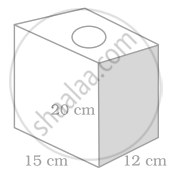Exercise | Q 101 | Page 357

A truck carrying 7.8 m3 concrete arrives at a job site. A platform of width 5 m and height 2 m is being contructed at the site. Find the length of the platform, constructed from the amount of concrete on the truck?

Exercise | Q 102 | Page 357

A hollow garden roller of 42 cm diameter and length 152 cm is made of cast iron 2 cm thick. Find the volume of iron used in the roller.

Exercise | Q 103 | Page 357

Three cubes each of side 10 cm are joined end to end. Find the surface area of the resultant figure.

Exercise | Q 104 | Page 357

Below are the drawings of cross sections of two different pipes used to fill swimming pools. Figure A is a combination of 2 pipes each having a radius of 8 cm. Figure B is a pipe having a radius of 15 cm. If the force of the flow of water coming out of the pipes is the same in both the cases, which will fill the swimming pool faster?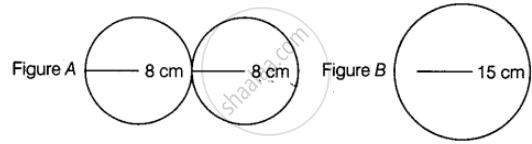Exercise | Q 105 | Page 257

A swimming pool is 200 m by 50 m and has an average depth of 2 m. By the end of a summer day, the water level drops by 2 cm. How many cubic metres of water is lost on the day?

Exercise | Q 106 | Page 358

A housing society consisting of 5,500 people needs 100 L of water per person per day. The cylindrical supply tank is 7 m high and has a diameter 10 m. For how many days will the water in the tank last for the society?

Exercise | Q 107 | Page 358

Metallic discs of radius 0.75 cm and thickness 0.2 cm are melted to obtain 508.68 cm3 of metal. Find the number of discs melted (use π = 3.14).

Exercise | Q 108 | Page 358

The ratio of the radius and height of a cylinder is 2:3. If its volume is 12,936 cm3, find the total surface area of the cylinder.

Exercise | Q 109 | Page 358

External dimensions of a closed wooden box are in the ratio 5:4:3. If the cost of painting its outer surface at the rate of Rs 5 per dm2 is Rs 11,750, find the dimensions of the box.

Exercise | Q 110 | Page 358

The capacity of a closed cylindrical vessel of height 1 m is 15.4 L. How many square metres of metal sheet would be needed to make it?

Exercise | Q 111.(a) | Page 358

What will happen to the volume of the cube, if its edge is tripled?

Exercise | Q 111.(b) | Page 358

What will happen to the volume of the cube, if its edge is reduced to one-fourth?

Exercise | Q 112 | Page 358

A rectangular sheet of dimensions 25 cm × 7 cm is rotated about its longer side. Find the volume and the whole surface area of the solid thus generated.

Exercise | Q 113 | Page 358

From a pipe of inner radius 0.75 cm, water flows at the rate of 7 m per second. Find the volume in litres of water delivered by the pipe in 1 hour.

Exercise | Q 114 | Page 358

Four times the area of the curved surface of a cylinder is equal to 6 times the sum of the areas of its bases. If its height is 12 cm, find its curved surface area.

Exercise | Q 115 | Page 358

A cylindrical tank has a radius of 154 cm. It is filled with water to a height of 3 m. If water to a height of 4.5 m is poured into it, what will be the increase in the volume of water in kl?

Exercise | Q 116 | Page 358

The length, breadth and height of a cuboidal reservoir is 7 m, 6 m and 15 m respectively. 8400 L of water is pumped out from the reservoir. Find the fall in the water level in the reservoir.

Exercise | Q 117 | Page 358

How many bricks of size 22 cm × 10 cm × 7 cm are required to construct a wall 11 m long, 3.5 m high and 40 cm thick, if the cement and sand used in the construction occupy (1/10)th part of the wall?

Exercise | Q 118 | Page 359

A rectangular examination hall having seats for 500 candidates has to be built so as to allow 4 cubic metres of air and 0.5 square metres of floor area per candidate. If the length of hall be 25 m, find the height and breadth of the hall.

Exercise | Q 119 | Page 359

The ratio between the curved surface area and the total surface area of a right circular cylinder is 1:2. Find the ratio between the height and radius of the cylinder.

Exercise | Q 120 | Page 359

A birthday cake has two tiers as shown in the figure below. Find the volume of the cake.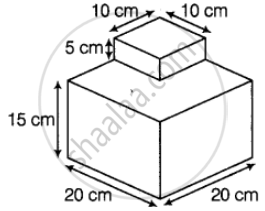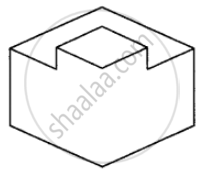Exercise | Q 121 | Page 359

Work out the surface area of following shapes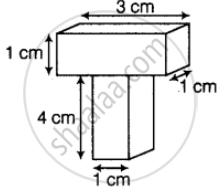Exercise | Q 122 | Page 359

Work out the surface area of following shapes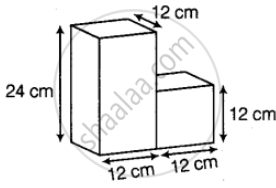Exercise | Q 123 | Page 359

Work out the surface area of following shapes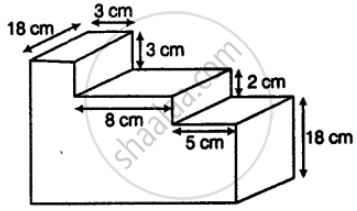Exercise | Q 124 | Page 359

Work out the surface area of following shapes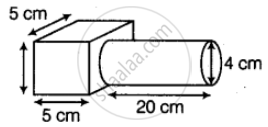Exercise | Q 125 | Page 359

Water flows from a tank with a rectangular base measuring 80 cm by 70 cm into another tank with a square base of side 60 cm. If the water in the first tank is 45 cm deep, how deep will it be in the second tank?

Exercise | Q 126 | Page 360

A rectangular sheet of paper is rolled in two different ways to form two different cylinders. Find the volume of cylinders in each case if the sheet measures 44 cm × 33 cm.

## Chapter 11: Mensuration

Exercise## NCERT solutions for Mathematics Exemplar Class 8 chapter 11 - Mensuration

NCERT solutions for Mathematics Exemplar Class 8 chapter 11 (Mensuration) include all questions with solution and detail explanation. This will clear students doubts about any question and improve application skills while preparing for board exams. The detailed, step-by-step solutions will help you understand the concepts better and clear your confusions, if any. Shaalaa.com has the CBSE Mathematics Exemplar Class 8 solutions in a manner that help students grasp basic concepts better and faster.

Further, we at Shaalaa.com provide such solutions so that students can prepare for written exams. NCERT textbook solutions can be a core help for self-study and acts as a perfect self-help guidance for students.

Concepts covered in Mathematics Exemplar Class 8 chapter 11 Mensuration are Area of Trapezium, Area of a General Quadrilateral, Area of a Polygon, Plane Figures and Solid Shapes, Mensuration, Area of a Rhombus, Surface Area of a Cube, Surface Area of a Cuboid, Surface Area of Cylinder, Concept of Surface Area, Volume, and Capacity, Volume of a Cuboid, Volume of Cube, Volume of a Cylinder.

Using NCERT Class 8 solutions Mensuration exercise by students are an easy way to prepare for the exams, as they involve solutions arranged chapter-wise also page wise. The questions involved in NCERT Solutions are important questions that can be asked in the final exam. Maximum students of CBSE Class 8 prefer NCERT Textbook Solutions to score more in exam.

Get the free view of chapter 11 Mensuration Class 8 extra questions for Mathematics Exemplar Class 8 and can use Shaalaa.com to keep it handy for your exam preparation